NEET  >  DC Pandey Solutions: Superposition of Waves - 1

# DC Pandey Solutions: Superposition of Waves - 1 - Physics Class 11 - NEET

## Introductory Exercise 15.1

Q.1. A string vibrates according to the equation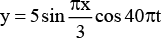where x and y are in centimetres and t is in seconds.
(a) What is the speed of the component wave?
(b) What is the distance between the adjacent nodes?
(c) What is the velocity of the particle of the string at the position x = 1.5 cm when t = 9/8 s?

Sol.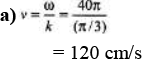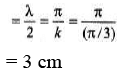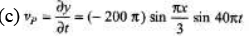Now substitute the given values of x and t.

Q.2. If two waves differ only in amplitude and are propagated in opposite directions through a medium, will they produce standing waves? Is energy transported?
Sol.
In this case, node points will also oscillate, but standing waves are formed.

Ques 3: Figure shows different standing wave patterns on a string of linear mass density 4.0 × 10-2 kg/m under a tension of 100 N. The amplitude of antinodes is indicated in each figure. The length of the string is 2.0 m.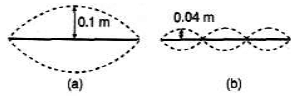(i) Obtain the frequencies of the modes shown in figures (a) and (b).

(ii) Write down the transverse displacement y as a function of x and f for each mode. (Take the initial configuration of the wire in each mode to be as shown by the dark lines in the figure).
Sol: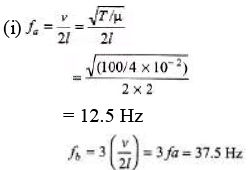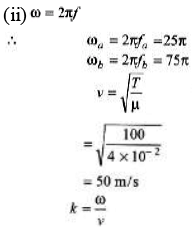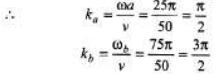Q.4. A 160 g rope 4 m long is fixed at one end and tied to a light string of the same length at the other end. Its tension is 400 N.
(a) What are the wavelengths of the fundamental and the first two overtones?
(b) What are the frequencies of these standing waves?
[Hint: In this case, the fixed end is a node and the end tied with the light string is antinode.]
Sol.

(a) 1 = λ1/4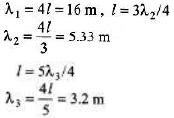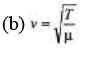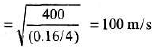Now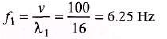Similarly f2 and f3.

Q.5. A string fastened at both ends has successive resonances with wavelengths of 0.54m for the nth harmonic and 0.48 m for the (n +1)th harmonic.
(a) Which harmonics are these?
(b) What is the length of the string?
(c) What is the wavelength of the fundamental frequency?
Sol.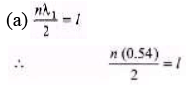or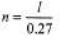...(i)
Similarly,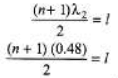or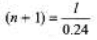... (ii)
From Eqs. (i) and (ii) we have,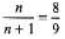(b) From Eq. (i)
l = (0.27)n = 0.27 × 8 = 2.16 m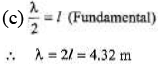Q.6. If the frequencies of the second and fifth harmonics of a string differ by 54 Hz. What is the fundamental frequency of the string?
Sol.

5f - 2f=54
3f = 54 f = 18 Hz

Q.7. A wire is attached to a pan of mass 200 g that contains a 2.0 kg mass, as shown in the figure. When clucked, the wire vibrates at a fundamental frequency of 220 Hz. An additional unknown mass M is then added to the pan and a fundamental frequency of 260 Hz is detected. What is the value of M1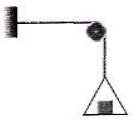Sol.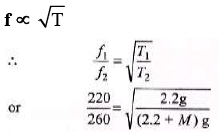Solving we get,
M = 0.873 kg

Q.8. A wire fixed at both ends is 1.0 m long and has a mass of 36 g. One of its oscillation frequencies is 250 Hz and the next higher one is 300 Hz.
(a) Which harmonics do these frequencies represent?
(b) What is the tension in the wire?
Sol.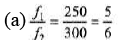So, f1 is 5th harmo nic and f2 is 6th harmonic.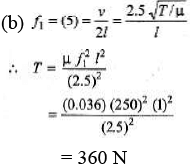The document DC Pandey Solutions: Superposition of Waves - 1 | Physics Class 11 - NEET is a part of the NEET Course Physics Class 11.
All you need of NEET at this link: NEET

## FAQs on DC Pandey Solutions: Superposition of Waves - 1 - Physics Class 11 - NEET

 1. What is the concept of superposition of waves?Ans. The concept of superposition of waves states that when two or more waves meet at a point in space, the resultant displacement at that point is the algebraic sum of the individual displacements produced by each wave.
 2. How can superposition of waves be used to analyze interference patterns?Ans. Superposition of waves can be used to analyze interference patterns by considering the constructive and destructive interference between two or more waves. Constructive interference occurs when the crests of two waves coincide, resulting in an increased amplitude, while destructive interference occurs when the crest of one wave coincides with the trough of another, resulting in a decreased amplitude.
 3. What is the principle of superposition and how does it apply to wave interference?Ans. The principle of superposition states that when two or more waves meet at a point, the resulting displacement is the vector sum of the individual displacements. In the context of wave interference, this principle explains how multiple waves can combine to create regions of constructive and destructive interference.
 4. Can waves of different frequencies superpose and interfere with each other?Ans. Yes, waves of different frequencies can superpose and interfere with each other. When waves of different frequencies meet, their superposition can result in complex interference patterns. These patterns can be observed in phenomena such as beats, where two waves of slightly different frequencies interfere to create a periodic variation in amplitude.
 5. How does the superposition of waves relate to the phenomenon of diffraction?Ans. The superposition of waves plays a key role in the phenomenon of diffraction. When a wave encounters an obstacle or passes through a narrow opening, it undergoes diffraction, which is the bending and spreading of the wave around the edges of the obstacle or opening. This bending and spreading occur due to the superposition of waves from different parts of the wavefront.

## Physics Class 11

130 videos|472 docs|210 tests

## Physics Class 11

130 videos|472 docs|210 tests

### How to Prepare for NEET

Read our guide to prepare for NEET which is created by Toppers & the best Teachers
Signup to see your scores go up within 7 days! Learn & Practice with 1000+ FREE Notes, Videos & Tests.
10M+ students study on EduRev
Track your progress, build streaks, highlight & save important lessons and more!(Scan QR code)
Related Searches

,

,

,

,

,

,

,

,

,

,

,

,

,

,

,

,

,

,

,

,

,

;